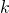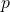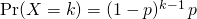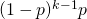# Metaphysics on geometric distribution in probability theory

I realized geometric distribution is not exactly about the time needed to get the first success in a given number of trials. This is a very odd feeling. It is probably a feeling applied mathematicians get sometimes, when they feel they are doing the best they can, and yet the theory is not perfect.

This may be a naive post, I warn you, but I was really stunned when I realized this.

### Geometric distribution is not about the first success

Let’s jump to the point. We know (or at least, I was taught) that geometric distribution is used to calculate the probability that the first success intrials (all independent and of probability) will happen precisely at the-th trial.

Remember that a geometric distribution is a random variablesuch that its distribution isHow can we relate the above distribution with the fact that it matches the first success? Well, we need to have one success, which explains theat the bottom. Moreover, we want to have just one success, so all other trials must be unsuccessful, which explains the.

But hey, where would first ever be written? Unless you do probability in a non-commutative ring (in which case, I don’t know what you are doing), multiplication is commutative. So who can tell the order between the events in a Bernoulli process?

In fact,could just as well refer to having unsuccessful outcomes for the firsttrials and then a successful one at the-th trial, as to having a success in the very first attempt and then all failures. As it is, as long as we have one (and only one) success among theattempts, the geometric distribution holds!

Apparently then, geometric distribution is about the time of first success, but it is not just about that. It encompasses way more cases, all equally likely. Geometric distribution allows to calculate exactly one success will happen intrials in a Bernoulli process.

The universe does not care about the order of events (in a Bernoulli process, at least). As long as we dotrials, regardless of when the success happens, the universe does not care. This stuns me!